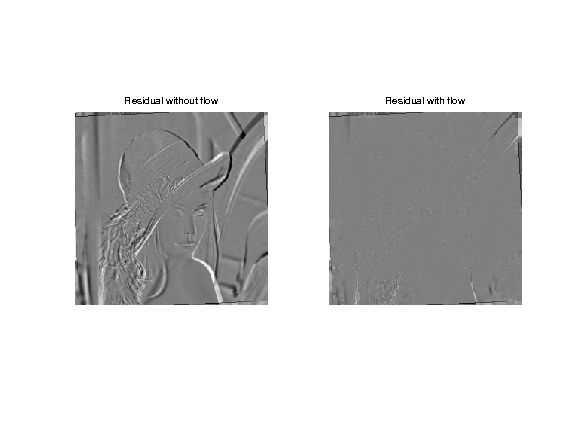# Optical Flow Computation¶

Important: Please read the installation page for details about how to install the toolboxes. $\newcommand{\dotp}{\langle #1, #2 \rangle}$ $\newcommand{\enscond}{\lbrace #1, #2 \rbrace}$ $\newcommand{\pd}{ \frac{ \partial #1}{\partial #2} }$ $\newcommand{\umin}{\underset{#1}{\min}\;}$ $\newcommand{\umax}{\underset{#1}{\max}\;}$ $\newcommand{\umin}{\underset{#1}{\min}\;}$ $\newcommand{\uargmin}{\underset{#1}{argmin}\;}$ $\newcommand{\norm}{\|#1\|}$ $\newcommand{\abs}{\left|#1\right|}$ $\newcommand{\choice}{ \left\{ \begin{array}{l} #1 \end{array} \right. }$ $\newcommand{\pa}{\left(#1\right)}$ $\newcommand{\diag}{{diag}\left( #1 \right)}$ $\newcommand{\qandq}{\quad\text{and}\quad}$ $\newcommand{\qwhereq}{\quad\text{where}\quad}$ $\newcommand{\qifq}{ \quad \text{if} \quad }$ $\newcommand{\qarrq}{ \quad \Longrightarrow \quad }$ $\newcommand{\ZZ}{\mathbb{Z}}$ $\newcommand{\CC}{\mathbb{C}}$ $\newcommand{\RR}{\mathbb{R}}$ $\newcommand{\EE}{\mathbb{E}}$ $\newcommand{\Zz}{\mathcal{Z}}$ $\newcommand{\Ww}{\mathcal{W}}$ $\newcommand{\Vv}{\mathcal{V}}$ $\newcommand{\Nn}{\mathcal{N}}$ $\newcommand{\NN}{\mathcal{N}}$ $\newcommand{\Hh}{\mathcal{H}}$ $\newcommand{\Bb}{\mathcal{B}}$ $\newcommand{\Ee}{\mathcal{E}}$ $\newcommand{\Cc}{\mathcal{C}}$ $\newcommand{\Gg}{\mathcal{G}}$ $\newcommand{\Ss}{\mathcal{S}}$ $\newcommand{\Pp}{\mathcal{P}}$ $\newcommand{\Ff}{\mathcal{F}}$ $\newcommand{\Xx}{\mathcal{X}}$ $\newcommand{\Mm}{\mathcal{M}}$ $\newcommand{\Ii}{\mathcal{I}}$ $\newcommand{\Dd}{\mathcal{D}}$ $\newcommand{\Ll}{\mathcal{L}}$ $\newcommand{\Tt}{\mathcal{T}}$ $\newcommand{\si}{\sigma}$ $\newcommand{\al}{\alpha}$ $\newcommand{\la}{\lambda}$ $\newcommand{\ga}{\gamma}$ $\newcommand{\Ga}{\Gamma}$ $\newcommand{\La}{\Lambda}$ $\newcommand{\si}{\sigma}$ $\newcommand{\Si}{\Sigma}$ $\newcommand{\be}{\beta}$ $\newcommand{\de}{\delta}$ $\newcommand{\De}{\Delta}$ $\newcommand{\phi}{\varphi}$ $\newcommand{\th}{\theta}$ $\newcommand{\om}{\omega}$ $\newcommand{\Om}{\Omega}$

This numerical tour explores the computation of optical flow between two images. It is at the heart of video coding.

In :
addpath('toolbox_signal')


To evaluate the performance of optical flow computation, we compute a pair of image obtained by a smooth warping (small deformation), here a simple rotation.

Original frame #1.

In :
n = 256;
name = 'lena';


The second image |M2| is obtaind by rotating the first one.

angle of rotation

In :
theta = .03 * pi/2;


original coordinates

In :
[Y,X] = meshgrid(1:n,1:n);


rotated coordinates

In :
X1 = (X-n/2)*cos(theta) + (Y-n/2)*sin(theta) + n/2;
Y1 =-(X-n/2)*sin(theta) + (Y-n/2)*cos(theta) + n/2;


boundary handling

In :
X1 = mod(X1-1,n)+1;
Y1 = mod(Y1-1,n)+1;


interpolation

In :
M2 = interp2(Y,X,M1,Y1,X1);
M2(isnan(M2)) = 0;


Display the two images.

In :
clf;
imageplot(M1, 'Frame #1', 1,2,1);
imageplot(M2, 'Frame #2', 1,2,2);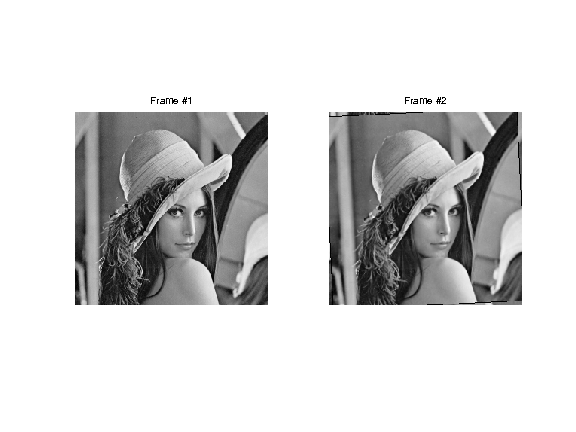## Optical Flow Computation with Regularization¶

A first approach to optical flow computation is to solve a ill posed problem corresponding to the optical flow equation constraint (consistency of gray level intensity when moving along the flow).

Compute the derivatives in time and space.

In :
global D;
Dt = M1-M2;


Display them.

In :
clf;
imageplot(Dt, 'd/dt', 1,3,1);
imageplot(D(:,:,1), 'd/dx', 1,3,2);
imageplot(D(:,:,2), 'd/dy', 1,3,3);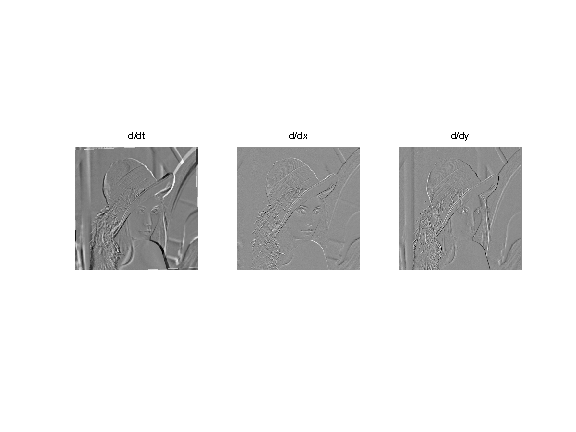The optical flow constraint asks for consistency of gray levels when moving along the flow |v=[v1,v2]|. This is written as a linear equation

|Dt + v1.D1 + v2.D2=0|

This equation does not constrain enough the flow (one equation for two unknown). One thus needs to add other constraints, and this is achieved by performing a Sobolev regularization, as first proposed by Horn and Schunck in the paper:

Horn, B.K.P., and Schunck, B.G., Determining Optical Flow, AI(17), No. 1-3, August 1981, pp. 185-203

This corresponds to a quadratic regularization with a Sobolev prior:

Its solution is computed by solving a linear system resolution, which sets to zero the gradient of the functional. It can be computed using a gradient descent, or, better, a conjugate gradient descent. We first detail the gradient descent, and shows that is not very efficient.

Regularization strength.

In :
global lambda;
lambda = .1;


In :
tau = .2;


Initialization.

In :
v = zeros(n,n,2);


Compute the gradient of the functional. First compute |Dt+v1D1+v2D2|

In :
U = Dt + sum(v.*D,3);


Then compute the Laplacian |L(:,:,k)| of each channel |v(:,:,k)| of the vector field

In :
L = cat(3, div(grad(v(:,:,1))), div(grad(v(:,:,2))));


And gather everything together to build the gradient of the functional.

In :
G = D.*repmat(U, [1 1 2])  - lambda * L;


Perform the descent.

In :
v = v - tau*G;


Exercise 1

Perform the gradient descent of the energy, and display the decay of the energy.

In :
exo1()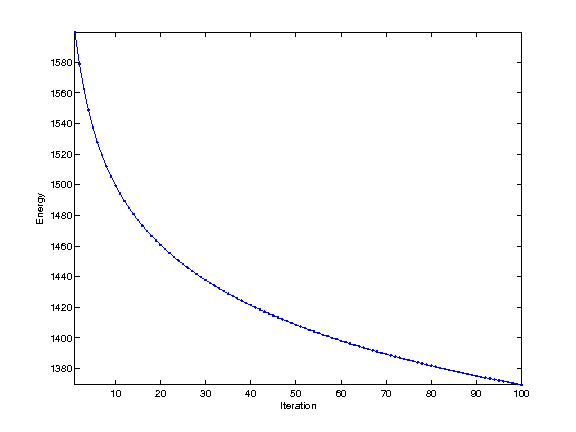In :
%% Insert your code here.


A much faster algorithm is the conjugate gradient. Several variant are implemented within matlab, and can be used by implementing a callback function.

Set up parameters for the CG algorithm (tolerance and maximum number of iterations.

In :
tol = 1e-5;
maxit = 200;


Right hand side of the linear system.

In :
b = -D.*cat(3,Dt,Dt);


In :
[v,flag,relres,it,resvec] = cgs(@callback_optical_flow,b(:),tol,maxit);
v = reshape(v, [n n 2]);


Display the flow as a color image and as arrows.

In :
clf;
imageplot(v, '', 1,2,1);
subplot(1,2,2);
w = 12; m = ceil(n/w);
t = w/2 + ((0:m-1)*w);
[V,U] = meshgrid(t,t);
hold on;
imageplot(M1);
quiver(t,t,v(1:w:n,1:w:n,2), v(1:w:n,1:w:n,1));
axis('ij');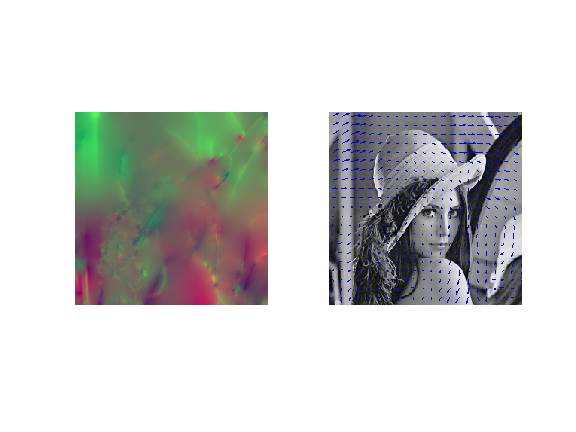Compute the image warped along the flow.

compute the grid, translated along the flow

In :
[Y,X] = meshgrid(1:n,1:n);
X = clamp(X+v(:,:,1),1,n);
Y = clamp(Y+v(:,:,2),1,n);


compute the first fame, translated along the flow

In :
Ms = interp2( 1:n,1:n, M1, Y,X );


One can compare the residual with and without the flow

residual without flow

In :
R0 = M2-M1;


residual along the flow

In :
R = Ms-M1;


ensure same dynamic range (just for display)

In :
v = max( [max(abs(R0(:))) max(abs(R(:)))] );
R(1)=v; R(2)=-v; R0(1)=v; R0(2)=-v;


display

In :
clf;
imageplot(R0, 'Residual without flow', 1,2,1);
imageplot(R, 'Residual with flow', 1,2,2);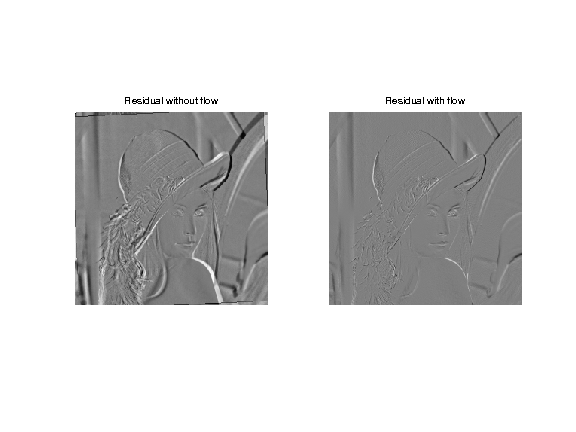## Optical Flow Computation with Block Matching¶

A second approach to compute the optical flow is to perform local block matching, as first proposed by Lucas and Kanade in

Lucas B D and Kanade T, An iterative image registration technique with an application to stereo vision Proceedings of Imaging understanding workshop, pp 121-130, 1981.

The advantage is that this is more precise than the global Horn/Schunck method, and it might also be faster (no iterative scheme is needed). The desadvantage is that it does not regularize the flow in flat region.

An optical flow is a vector field that describes the movement between to consecutive frames of the video.

The flow can be computed by block matching. A block of |(2k+1,2k+1)| pixels in frame 1 around a location |(x,y)| is compared to the blocks at locations |(x+dx,y+dy)| for |-q<=dy,dx<=q| in the frame 2.

width of the block

In :
w = 8;


search width

In :
q = 4;


sub-pixelic search if <1

In :
dq = .5;


Number of flow vector is |m^2|.

In :
m = ceil(n/w);


Precompute movements vectors.

In :
[X0,Y0,dX,dY] = ndgrid( 0:w-1, 0:w-1, -q:dq:q,-q:dq:q);
[dy,dx] = meshgrid(-q:dq:q,-q:dq:q);


Start with empty optical flow. Each |f=F(x,y,:)| is a 2D vector mapping the patch at location |(x,y)| to the patch |(x+f(1),y+f(2)|.

In :
F = zeros(n,n,2);


Example of block number for wich the flow is computed. Each index should be less than |m|

In :
i = 3; j = 40;


Pixel numbers.

In :
x = (i-1)*w+1;
y = (j-1)*w+1;


Block pixels index.

In :
selx = clamp( (i-1)*w+1:i*w, 1,n);
sely = clamp( (j-1)*w+1:j*w, 1,n);


A special care should be taken at the boundary : we simply clamp values outside boundaries

In :
X = clamp(x + X0 + dX,1,n);
Y = clamp(y + Y0 + dY,1,n);


Compute base patch of |M2| at which the flow is computed.

In :
P2 = M2(selx,sely);


Compute patches of |M1| that are matched. Use interpolation to handle non indeger pixel indexes.

In :
P1 = interp2( 1:n,1:n, M1, Y,X );


Compute the distance between |P1| and all the patches of |P2|.

In :
d = sum(sum( (P1-repmat(P2,[1 1 size(P1,3) size(P1,4)])).^2 ) );


Compute best match and report its value.

In :
[tmp,I] = compute_min(d(:));
F(selx,sely,1) = dx(I);
F(selx,sely,2) = dy(I);


Exercise 2

Compute the whole optical flow |F|, by cycling through the pixels.

In :
exo2()

In :
%% Insert your code here.


Display the flow as a color image and as arrows.

In :
clf;
imageplot(F, '', 1,2,1);
subplot(1,2,2);
t = w/2 + ((0:m-1)*w);
[V,U] = meshgrid(t,t);
hold on;
imageplot(M1);
quiver(t,t,F(1:w:n,1:w:n,2), F(1:w:n,1:w:n,1));
axis('ij');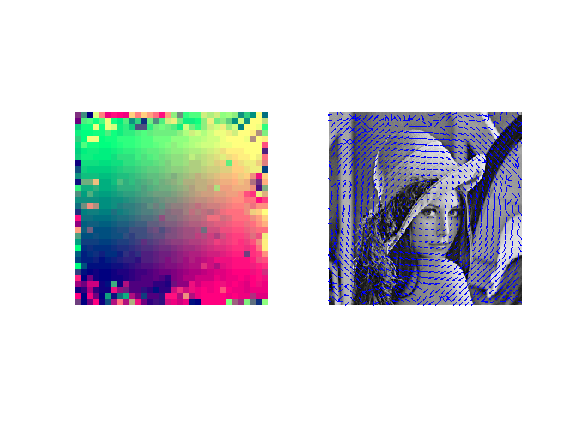## Residual Computation¶

The optical flow |F| allows one to compute the residual |R| between frame |M2| and an extrapolated version of |M1| along the flow |F|.

One can translate the first frame |M1| along the flow |F|.

compute the grid, translated along the flow

In :
[Y,X] = meshgrid(1:n,1:n);
X = clamp(X+F(:,:,1),1,n);
Y = clamp(Y+F(:,:,2),1,n);


compute the first fame, translated along the flow

In :
Ms = interp2( 1:n,1:n, M1, Y,X );


One can compare the residual with and without the flow

residual without flow

In :
R0 = M2-M1;


residual along the flow

In :
R = M2-Ms;


ensure same dynamic range (just for display)

In :
v = max( [max(abs(R0(:))) max(abs(R(:)))] );
R(1)=v; R(2)=-v; R0(1)=v; R0(2)=-v;


display

In :
clf;
imageplot(R0, 'Residual without flow', 1,2,1);
imageplot(R, 'Residual with flow', 1,2,2);